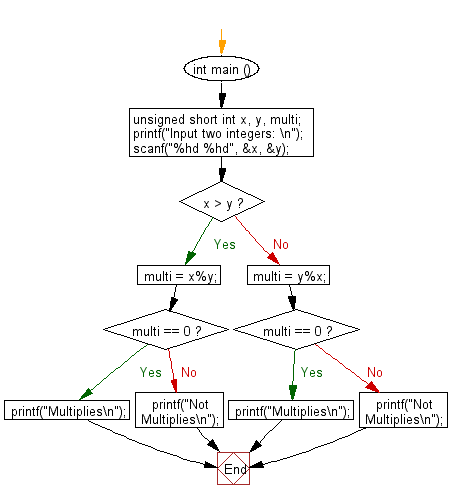﻿ C Program: Test two integers are multiply or not - w3resource

# C Exercises: Test two integers are multiply or not

## C Basic Declarations and Expressions: Exercise-103 with Solution

Write a C program that takes two integers and tests whether they are multiplied or not.

In science, a multiple is the product of any quantity and an integer. In other words, for the quantities a and b, we say that b is a multiple of a if b = na for some integer n, which is called the multiplier. If a is not zero, this is equivalent to saying that b/a is an integer.

Sample Solution:

C Code:

``````#include <stdio.h>
int main () {

// Declare unsigned short integer variables x, y, and multi
unsigned short int x, y, multi;

// Prompt the user to input two integers
printf("Input two integers: \n");

// Read the input values for x and y
scanf("%hd %hd", &x, &y);

// Check if x is greater than y
if (x > y){

// Calculate the remainder when x is divided by y
multi = x % y;

// Check if the remainder is zero
if ( multi == 0){
printf("Multiplies\n");
}
else{
printf("Not Multiplies\n");
}
}
else{
// Calculate the remainder when y is divided by x
multi = y % x;

// Check if the remainder is zero
if (multi == 0){
printf("Multiplies\n");
}
else{
printf("Not Multiplies\n");
}
}
}
``````

Sample Output:

```Input two integers:
3
9
Multiplies
```

Flowchart:C programming Code Editor:

What is the difficulty level of this exercise?

Test your Programming skills with w3resource's quiz.

﻿